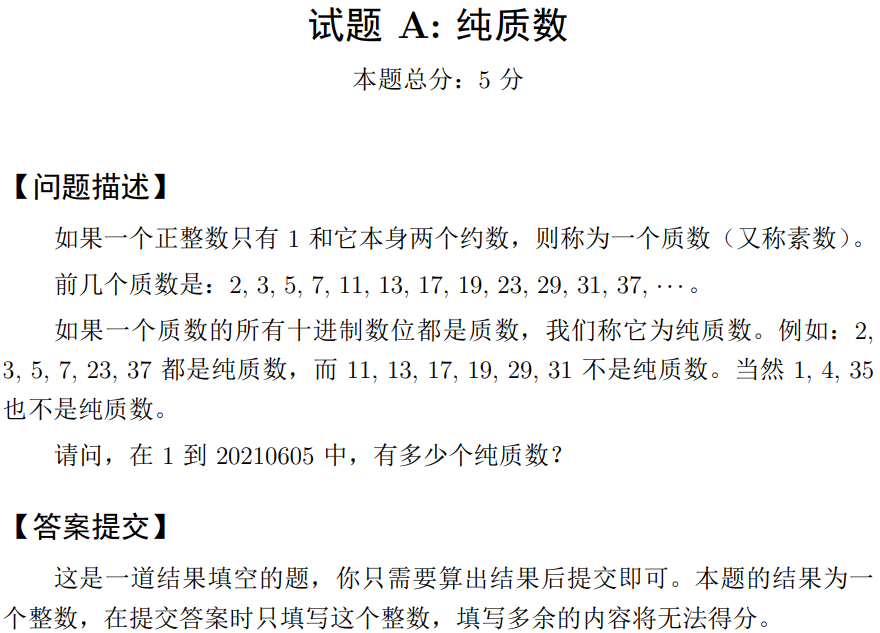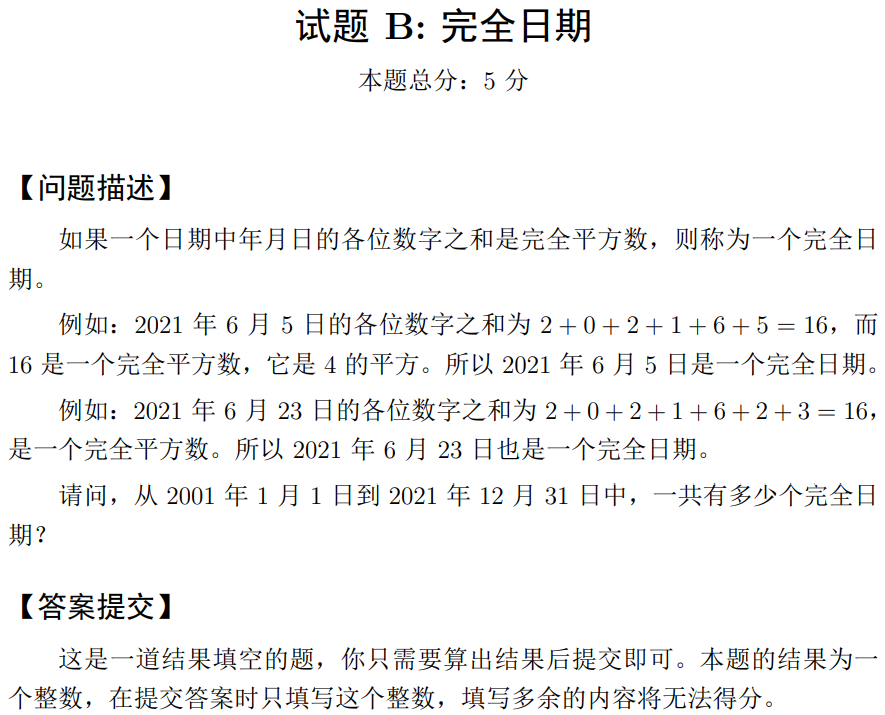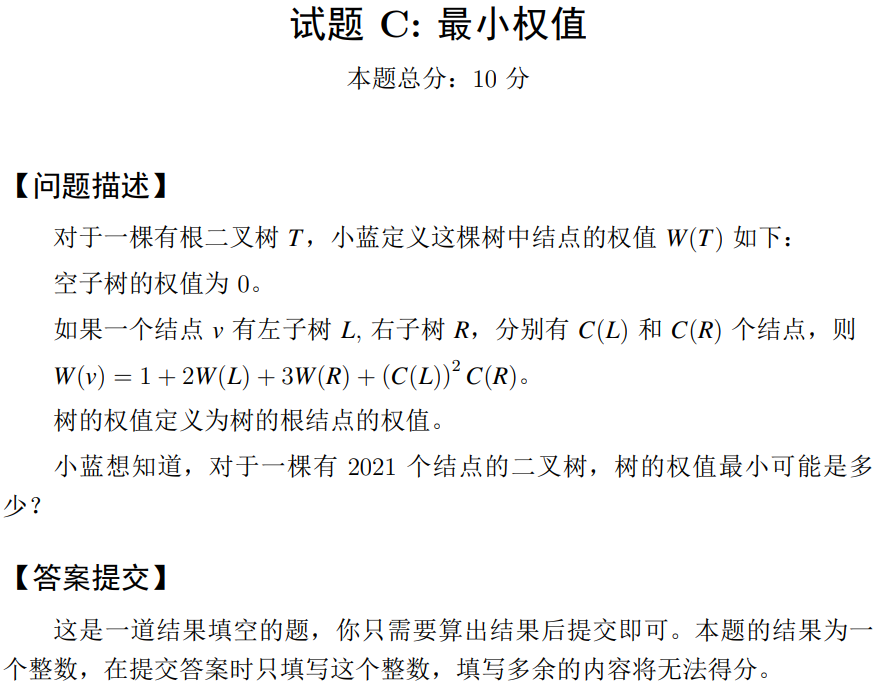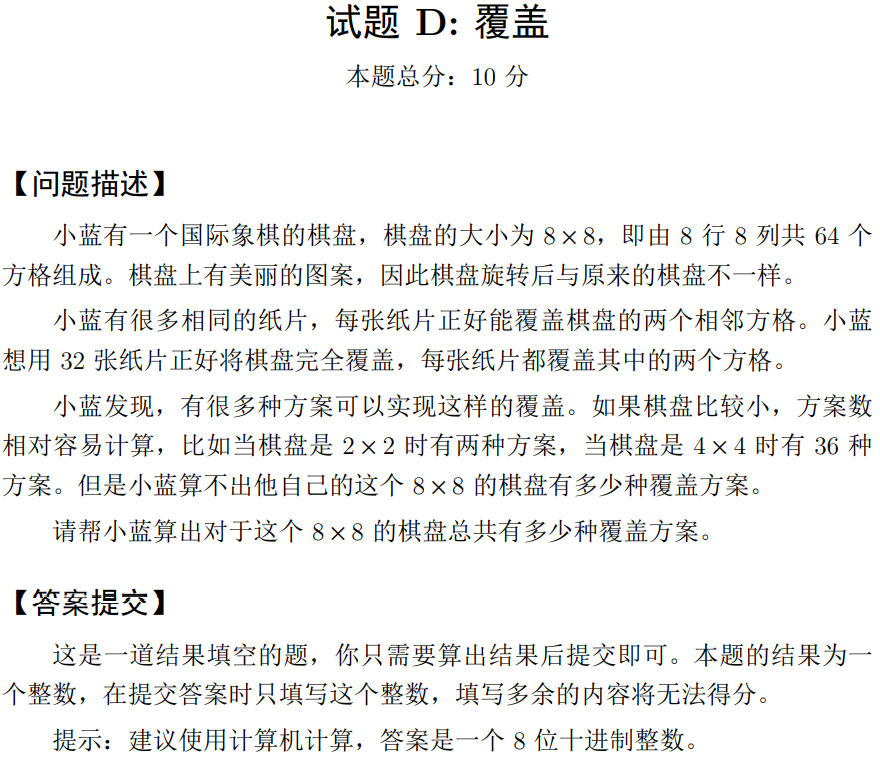@

## A题 纯质数（5分）#include <bits/stdc++.h>
using namespace std;
const int N=20210605;
bool judge(int x)
{
if(x==0||x==1)return 0;
for(int i=2;i*i<=x;i++)
if(x%i==0)return 0;
return 1;
}
int main()
{
ios::sync_with_stdio(false);
int ans=0;
for(int i=1;i<=N;i++)
{
if(judge(i))
{
int x=i;
bool flag=1;
while(x)
{
if(!judge(x%10)){flag=0;break;}
x/=10;
}
if(flag)ans++;
}
}
printf("%d\n",ans);
return 0;
}


## B题 完全日期（5分）#include <bits/stdc++.h>
using namespace std;
const int N=1e6+10;
bool vis[N];
int get_day(int y,int m)
{
if(m==2)
{
if(y%4==0&&y%100!=0)return 29; // 2020.02.29
else return 28; // 2021.02.28
}
else
{
if(m==1||m==3||m==5||m==7||m==8||m==10||m==12)return 31;
return 30;
}
}
int f(int x)
{
int s=0;
while(x)
{
s+=x%10;
x/=10;
}
return s;
}
int main()
{
for(int i=1;i<=1000;i++)
vis[i*i]=1;
int ans=0;
for(int y=2001;y<=2021;y++)
{
for(int m=1;m<=12;m++)
{
int allday=get_day(y,m);
for(int day=1;day<=allday;day++)
{
int sum=f(y)+f(m)+f(day);
if(vis[sum])ans++;
}
}
}
printf("%d\n",ans);
return 0;
}


## C题 最小权值（10分）## D题 覆盖（10分）## E题 123（15分）

试题 E: 123

【问题描述】

1, 1, 2, 1, 2, 3, 1, 2, 3, 4, ...

3 项是整数 1 至 3，接下来 4 项是整数 1 至 4，依次类推。

【输入格式】

【输出格式】

【样例输入】
3
1 1
1 3
5 8
【样例输出】
1
4
8
【评测用例规模与约定】



#include <bits/stdc++.h>
using namespace std;
typedef unsigned long long ll;
const ll N=2e6+3;
ll a[N+10],sum[N+10];
ll get_id(ll x) // 得到组号
{
return (ll)(lower_bound(a+1,a+N+1,x)-a);
}
int main()
{
ios::sync_with_stdio(false);
for(ll i=1;i<=N;i++)
{
a[i]=(1+i)*i/2; // 前i组个数
sum[i]=sum[i-1]+a[i]; // 前i组的和
}
int T;cin>>T;
ll l,r,ans;
while(T--)
{
cin>>l>>r;
ll n1=get_id(l);
ll n2=get_id(r);
if(n1==n2) // 同一组
{
ll num=r-l+1;
ll x=l-a[n1-1];
ans=x*num+num*(num-1)/2;
}
else
{
ll num=a[n1]-l+1;
ll x=l-a[n1-1];
ll s1=x*num+num*(num-1)/2; // 前面部分
ll s2=sum[n2-1]-sum[n1]; // 中间的完整组
num=r-a[n2-1];
ll s3=num+num*(num-1)/2; // 最后部分
ans=s1+s2+s3;
}
printf("%llu\n",ans);
}
return 0;
}
/*
999999999999 1000000000000
ans:176417
1000000000000 1000000000000
ans:88209
*/


## F题 异或变换（15分）

试题 F: 异或变换

【问题描述】

s1′ = s1;
si = si−1′ ⊕ si。

【输入格式】

【输出格式】

【样例输入】
5 3
10110
【样例输出】
11010
【样例说明】

【评测用例规模与约定】



#include <bits/stdc++.h>
using namespace std;
typedef long long ll;
unordered_map<string,ll>vis;
unordered_map<ll,string>mp;
string f(string s)
{
string res=s;
for(int i=1;s[i];i++)
res[i]=(s[i]-'0')^(s[i-1]-'0')+'0';
return res;
}
int main()
{
ios::sync_with_stdio(false);
string s;
int n;ll k;
cin>>n>>k>>s;
vis[s]=(ll)1;
mp[(ll)1]=s;
for(ll i=2;i<=k+1;i++) // k次
{
s=f(s);
if(!vis[s])
{
vis[s]=i;
mp[i]=s;
}
else // 找到循环节
{
ll pos=vis[s]; // 上一次出现的位置
ll ansk=(k+1-pos)%(i-pos);
s=mp[pos+ansk];
break;
}
}
printf("%s\n",s.c_str());
return 0;
}
/*
5 1000000000000000000
10110
*/


## G题 冰山（20分）

试题 G: 冰山

【问题描述】

Yi 的冰山（Yi = 0 表示没有冰山漂来）。

【输入格式】

, Yi，意义如前所述。
【输出格式】

【样例输入】
1 3 6
1
6 1
2 2
-1 1
【样例输出】
8
16
11
【样例说明】

【评测用例规模与约定】



#include <bits/stdc++.h>
using namespace std;
typedef long long ll;
const ll N=1e5+10,mod=998244353;
vector<ll>g;
vector<ll> f(vector<ll> t,ll x,ll y,ll k)
{
vector<ll>res;
ll sz=(ll)t.size();
for(ll i=0;i<sz;i++)
{
t[i]+=x;
if(t[i]>0) // 只保留大于0的
{
if(t[i]<=k)res.push_back(t[i]);
else // 分裂
{
res.push_back(k);
for(ll j=1;j<=t[i]-k;j++) // 没想到这里怎么优化
res.push_back((ll)1);
}
}
}
if(y>0)res.push_back(y);
return res;
}
int main()
{
ios::sync_with_stdio(false);
ll n,m,k,x,y;
cin>>n>>m>>k;
for(ll i=1;i<=n;i++)
{
cin>>x;
g.push_back(x);
}
for(ll i=1;i<=m;i++)
{
cin>>x>>y;
g=f(g,x,y,k);
ll sum=0;
for(ll j=0;j<g.size();j++)
sum=(sum+g[j])%mod;
printf("%lld\n",sum);
}
return 0;
}


## H题 翻转括号序列（20分）

试题 H: 翻转括号序列

【问题描述】

1. 将 [Li, Ri] 区间内（序列中的第 Li 个字符到第 Ri 个字符）的括号全部翻

2. 求出以 Li 为左端点时，最长的合法括号序列对应的 Ri （即找出最大的
Ri 使 [Li, Ri] 是一个合法括号序列）。
【输入格式】

【输出格式】

【样例输入】
7 5
((())()
2 3
2 2
1 3 5
2 3
2 1
【样例输出】
4
7
0
0
【评测用例规模与约定】



#include <bits/stdc++.h>
using namespace std;
const int N=1e6+10,inf=0x7f7f7f7f;
typedef long long ll;
int n,m;
char s[N];
int judge(char s[],int l)
{
stack<char>g;
while(g.size())g.pop();
int ans=0;
for(int i=l;i<=n;i++)
{
if(g.empty())g.push(s[i]);
else
{
if(g.top()=='('&&s[i]==')')g.pop();
else g.push(s[i]);
}
if(g.empty()) ans=i;
}
return ans;
}
int main()
{
ios::sync_with_stdio(false);
int opt,l,r;
cin>>n>>m>>s+1;
for(int i=1;i<=m;i++)
{
cin>>opt;
if(opt==1)
{
cin>>l>>r;
for(int i=l;i<=r;i++)
{
if(s[i]=='(')s[i]=')';
else s[i]='(';
}
}
else
{
cin>>l;
printf("%d\n",judge(s,l));
}
}
return 0;
}


## I题 异或三角（25分）

试题 I: 异或三角

【问题描述】

1. 1 ≤ a, b, c ≤ ni；
2. a ⊕ b ⊕ c = 0，其中 ⊕ 表示二进制按位异或；
3. 长度为 a, b, c 的三条边能组成一个三角形。
【输入格式】

【输出格式】

【样例输入】
2
6
114514
【样例输出】
6
11223848130
【评测用例规模与约定】



#include <bits/stdc++.h>
using namespace std;
typedef long long ll;
int main()
{
ios::sync_with_stdio(false);
int T;cin>>T;
while(T--)
{
ll n;cin>>n;
ll ans=0;
for(ll i=1;i<=n;i++)
{
for(ll j=1;j<=n;j++)
{
ll k=i^j;
if(k<=n&&k>=1&&i+j>k&&i+k>j&&k+j>i)ans++;
}
}
printf("%lld\n",ans);
}
return 0;
}


## J题 积木（25分）

试题 J: 积木

【问题描述】

【输入格式】

【输出格式】

998244353 的余数。
【样例输入 1】
5 1 1 2 2 5 3
【样例输出 1】
4
【样例输入 2】
233 5 1 8 100 215 3
【样例输出 2】
308810105
【评测用例规模与约定】

0 ≤ L ≤ R ≤ 40, 1 ≤ x < y ≤ n, 0 ≤ z ≤ 10^9。


#include <bits/stdc++.h>
using namespace std;
typedef long long ll;
const ll N=1e5+10,mod=998244353;
ll a[N],ans,n,w,l,r,x,y,z;
void dfs(ll i,ll u)
{
a[i]=u;
if(i==y)
{
if(a[i]==a[x]*z)ans++;
return;
}
for(ll h=a[i]+l;h<=a[i]+r;h++)
dfs(i+1,h);
}
int main()
{
ios::sync_with_stdio(false);
cin>>n>>w>>l>>r>>x>>y>>z;
dfs(1,w);
printf("%lld\n",ans%mod);
return 0;
}
/*
10 1 0 4 1 10 2
ans: 9
*/